Subject:
Geometry
Material Type:
Lesson Plan
Level:
Middle School
7
Provider:
Pearson
Tags:
Language:
English
Media Formats:
Text/HTML

# Gallery Problems Exercise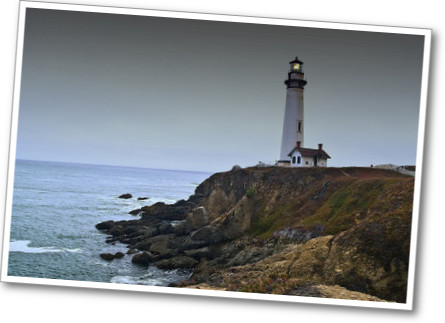## Overview

Allow students who have a clear understanding of the content thus far in the unit to work on Gallery problems of their choosing. You can then use this time to provide additional help to students who need review of the unit's concepts or to assist students who may have fallen behind on work.

# Gallery Problems

The SS Edmund Fitzgerald
Students solve mathematical problems about the sinking of the ship Edmund Fitzgerald.

Spirals
Students learn about the mathematics of spirals. They see spirals in nature and connect spirals to the Fibonacci.

Ship It!
Students learn about shipping containers and use a unit of measure that is only used in the shipping industry the twenty-foot equivalent unit (TEU).

Rideau Canal Waterway
Students compare information about the Rideau Canal and compare it with the Welland Canal.

A Rule of Thumb
Students learn about a “rule of thumb” that people use to estimate the speed of a train they are riding on. They investigate the mathematics of this rule.

Intersection
Students use information on a map to calculate where two streets will intersect.

Tolstoy's Problem
Students learn about Leo Tolstoy, a Russian writer who wrote two of the greatest novels of all time. They solve a problem that Tolstoy found very interesting.

The Dog Run
Students imagine having 22 meters of wire fencing for a dog run. They investigate how the area of the dog run changes as the length varies.

Bodies of Water
Students investigate a claim on the Runner's World website about the amount of water in the body of a 160-pound man.

# The SS Edmund Fitzgerald

1. Maximum dimensions for a Saint Lawrence Seaway ship: 740 feet by 78 feet.

11 feet shorter: 740 − 11 = 729 feet long

3 feet narrower: 78 − 3 = 75 feet wide

The dimensions of the Edmund Fitzgerald were 729 feet long by 75 feet wide.

2.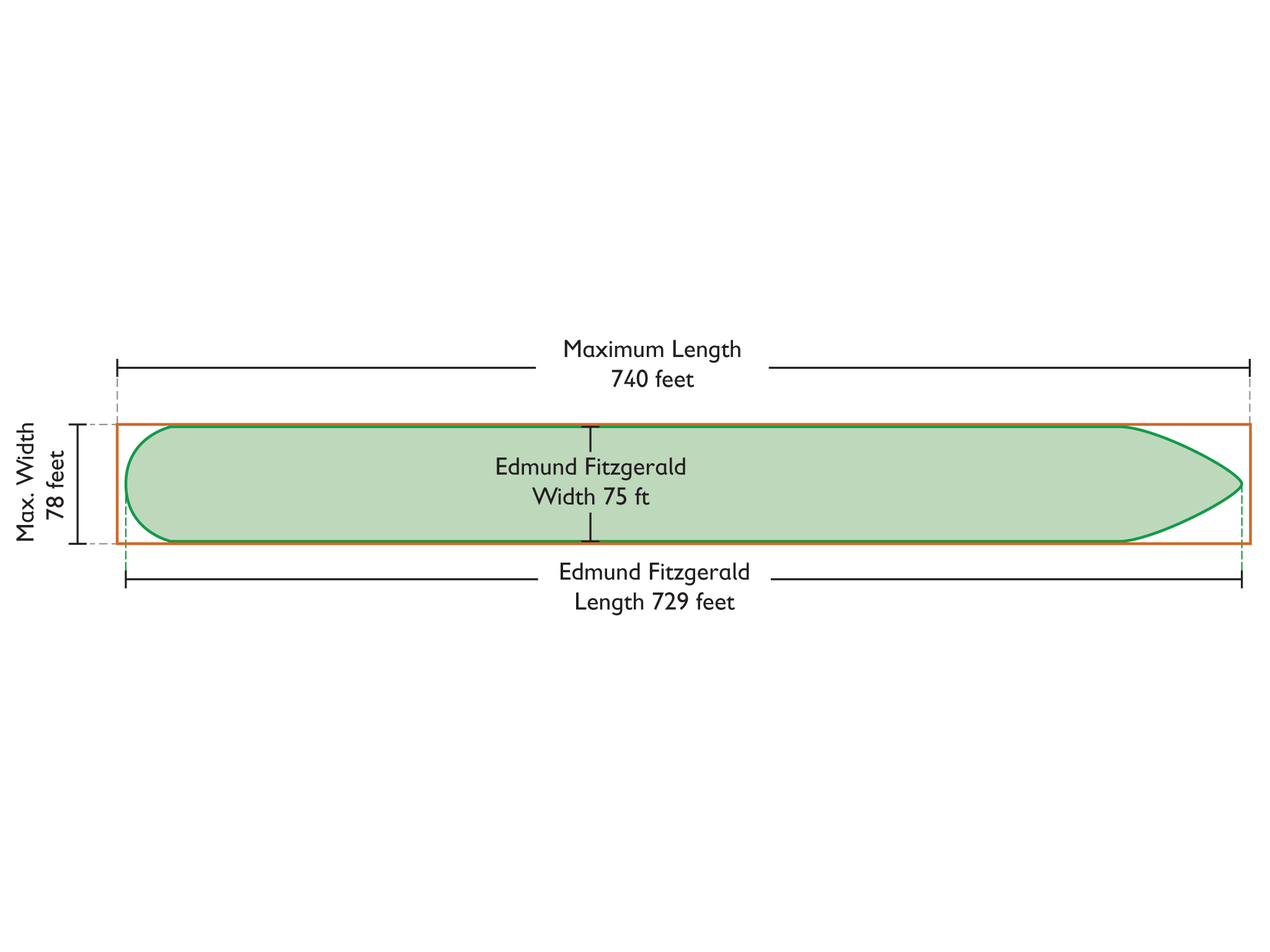3. The map distance between the dots for the ship's positions at 16:34 and 17:28 is 14 mm.

The time difference between these two positions is 54 minutes.

The map distance between the position at 17:28 and the wreckage position is 18 mm.

If x represents the time of sinking

$\begin{array}{c}\frac{54}{14}=\frac{x}{18}\left(\text{assuming}\text{\hspace{0.17em}}a\text{\hspace{0.17em}}\text{constant}\text{\hspace{0.17em}}\text{speed}\right).\\ \frac{54}{14}\text{is}\text{\hspace{0.17em}}\text{approximately}\text{\hspace{0.17em}}\text{3}\text{.86,}\text{\hspace{0.17em}}\text{and}\text{\hspace{0.17em}}\text{3}\text{.86}\text{\hspace{0.17em}}\text{•}\text{\hspace{0.17em}}\text{18=69}\text{.48}\text{.}\\ \text{Adding}\text{\hspace{0.17em}}\text{69}\text{.5}\text{\hspace{0.17em}}\text{minutes}\text{\hspace{0.17em}}\text{to}\text{\hspace{0.17em}}\text{17:28}\text{\hspace{0.17em}}\text{gives}\text{\hspace{0.17em}}\text{18:37}\text{.5,}\text{\hspace{0.17em}}\text{or}\text{\hspace{0.17em}}\text{about}\text{\hspace{0.17em}}\text{6:38}\text{\hspace{0.17em}}\text{pm}\text{.}\end{array}$

From the map data I estimate, the Edmund Fitzgerald sank at about 6:38 pm.

The map scale shows 1 mm: 1 statute mile.

Problem 3 showed that the ship traveled 14 mm (map scale) in 54 minutes, which is equal to 14 miles in 54 minutes.

If x represents the speed in miles per hour:

$\begin{array}{c}\frac{54\text{\hspace{0.17em}}\text{minutes}}{1}\cdot \frac{1\text{\hspace{0.17em}}\text{hour}}{60\text{\hspace{0.17em}}\text{minutes}}=0.9\text{\hspace{0.17em}}\text{hours}\\ \frac{14\text{\hspace{0.17em}}\text{miles}}{0.9\text{\hspace{0.17em}}\text{hours}}=\frac{x\text{\hspace{0.17em}}\text{miles}}{1\text{\hspace{0.17em}}\text{hour}}\\ 15.6\text{\hspace{0.17em}}\frac{\text{miles}}{\text{hour}}\approx x\end{array}$

From the map I estimate, the Edmund Fitzgerald's speed was about 15.6 miles per hour.

The map scale shows 50 mm: 80 km, or 1 mm: $\frac{80}{50}\text{km}$, or 1 mm: 1.6 km

Problem 3 showed the ship traveled 14 mm (map scale) in 54 minutes.

This distance is equal to 14 • 1.6 = 22.4 km in 54 minutes.

If x represents the speed in kilometers per hour:

$\begin{array}{c}\frac{22.4}{54}=\frac{x}{60}\\ \frac{22.4}{6•9}=\frac{x}{6•10}\\ \frac{22.4}{9}=\frac{x}{10}\\ \frac{22.4•10}{9}=\frac{x•10}{10}\\ \frac{224}{9}=x\\ x=\frac{224}{9}\approx 24.9\end{array}$

From the map I estimate, the Edmund Fitzgerald's speed was about 25 km per hour.

# The SS Edmund Fitzgerald

The sinking of the Titanic made headlines all over the world, and it also was the subject of a famous movie. But in the United States, another disaster came close: the sinking of the SS Edmund Fitzgerald. The Edmund Fitzgerald was the largest ship that could sail the St. Lawrence Seaway. The ship’s christening was seen as an unlucky sign: The bottle of champagne used to christen the ship took three times to break—what some considered a very bad omen.

On November 10, 1975, the Edmund Fitzgerald entered a very bad storm, close to Whitefish Bay, and sunk suddenly. No distress signals were ever sent. The ship did not get a movie, but it did get a beautiful song, The Wreck of the Edmund Fitzgerald by Gordon Lightfoot. Watch the video for some background on the Edmund Fitzgerald.

The maximum length for a ship on the St. Lawrence Seaway is 740 feet and the maximum width is 78 feet. When launched in 1958, the Edmund Fitzgerald was the largest boat on the Great Lakes, and it remains the largest boat to have sunk here.

1. The “Titanic of the Great Lakes” or “Mighty Fitz” was 11 feet shorter and 3 feet less wide than the maximum dimensions allowed in the Seaway. It was the first ship built to these dimensions. What were the dimensions of the ship?
2. Draw a top view of the Edmund Fitzgerald to scale. Put a rectangle of the maximum dimensions around our drawing of the ship.

The map represents the ship’s voyage on November 10, 1975, the day of its final trip.
You see a black dot with 12:52, which is the ship at 12 hours 52 minutes on November 10, 1975. Then the ship travels and you see where it was at 13:59; 14:45; 16:34; 17:28 and finally the place of the wreckage.

3. Make an estimate for the time of sinking.
4. Make an estimate for the speed of the ship using the scale lines.VIDEO: The Edmund Fitzgerald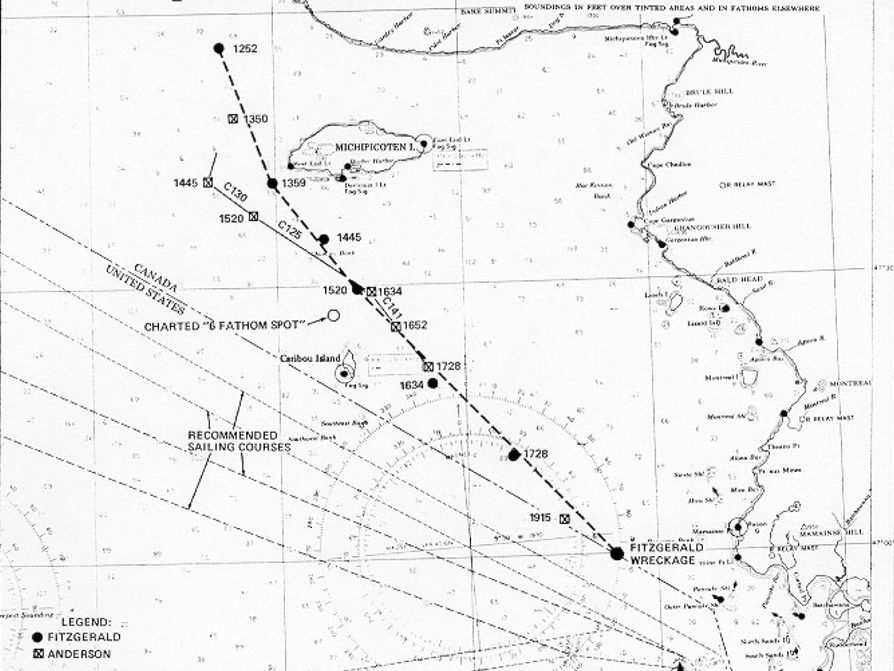# Spirals

1. The third rectangle has dimensions 3 wide by 2 tall; the fourth has dimensions 3 wide by 5 tall.
2. Fifth figure, which has dimensions 8 wide by 5 tall: Sixth figure, which has dimensions 8 wide by 13 tall: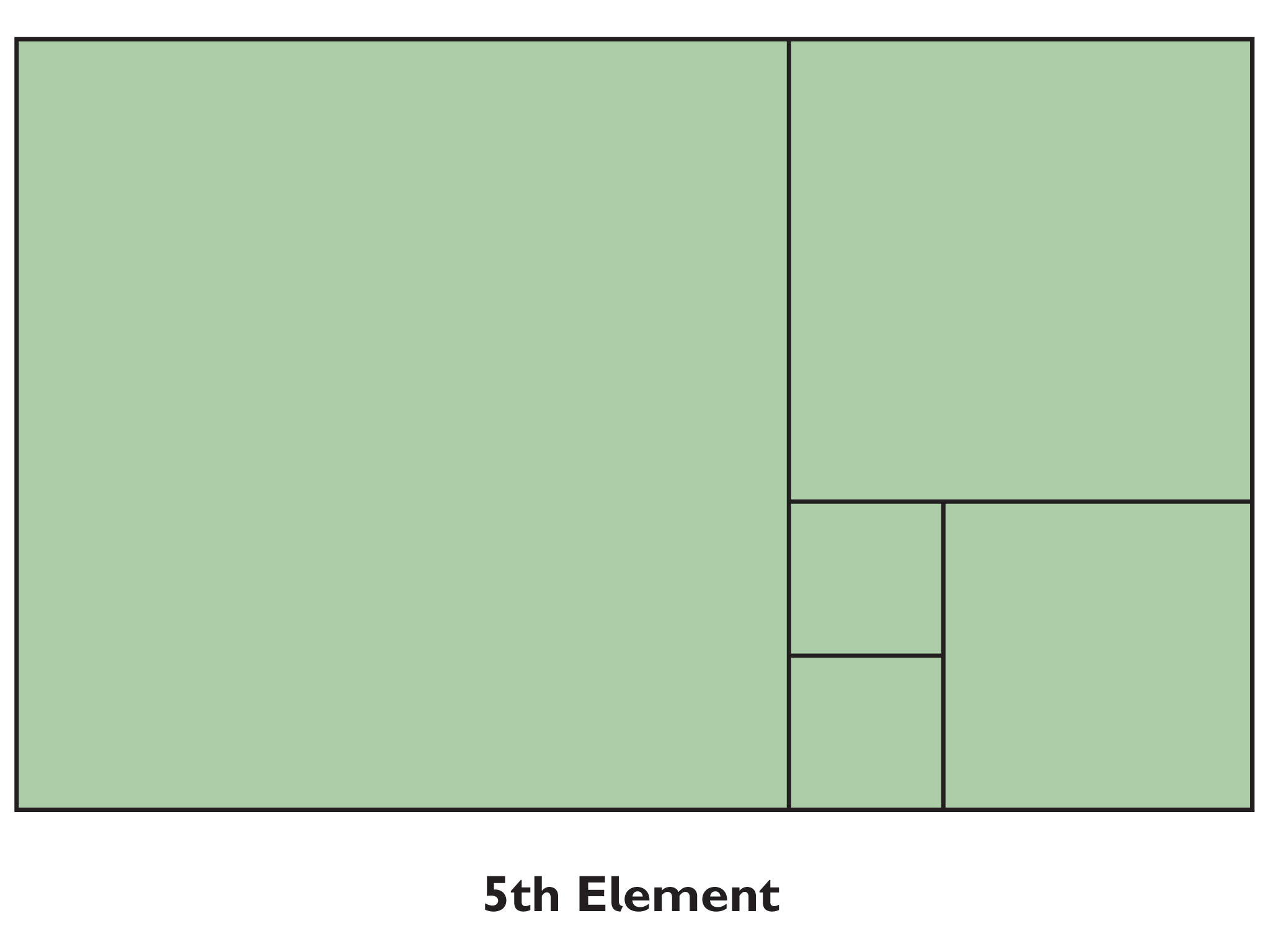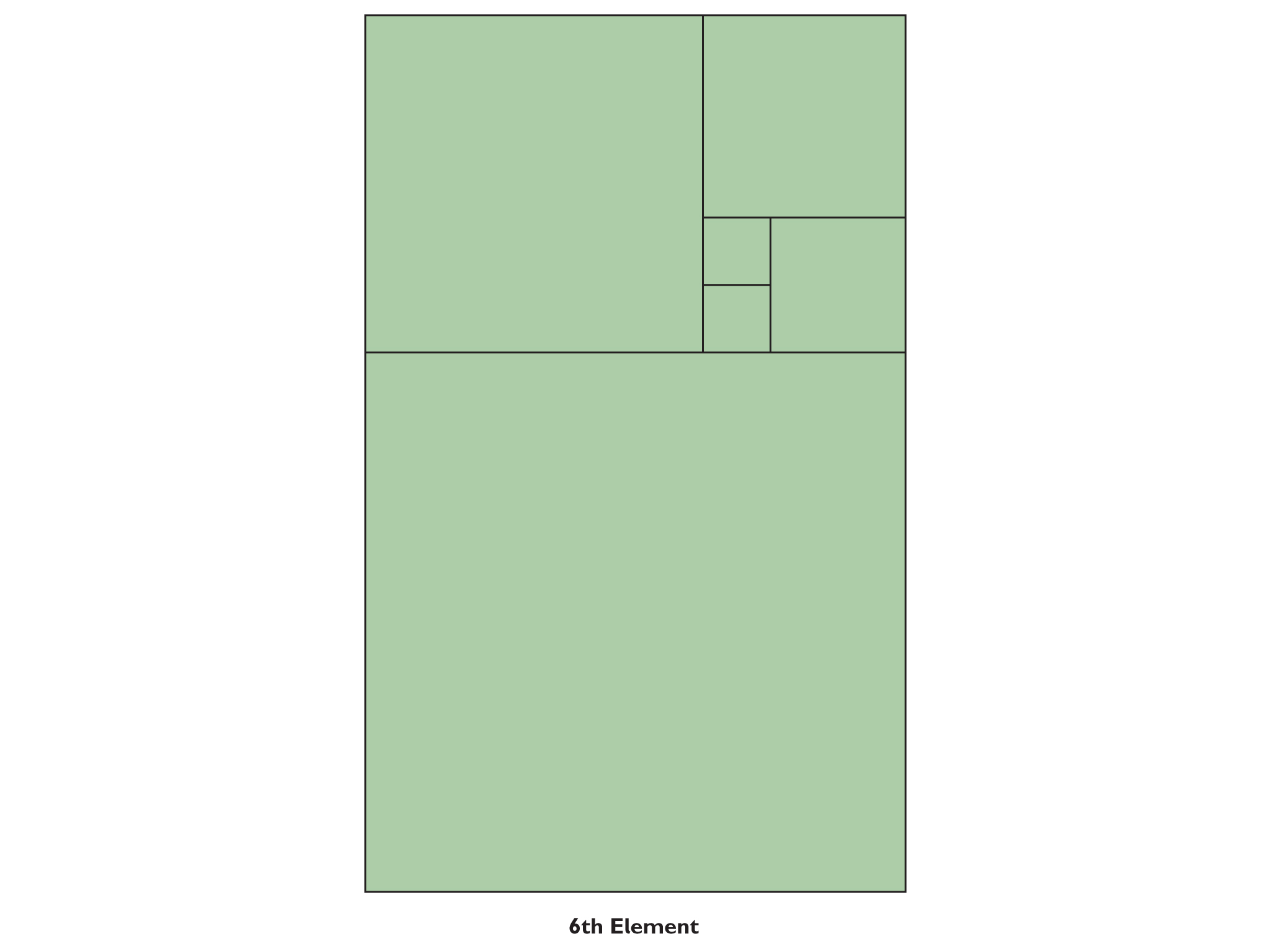3.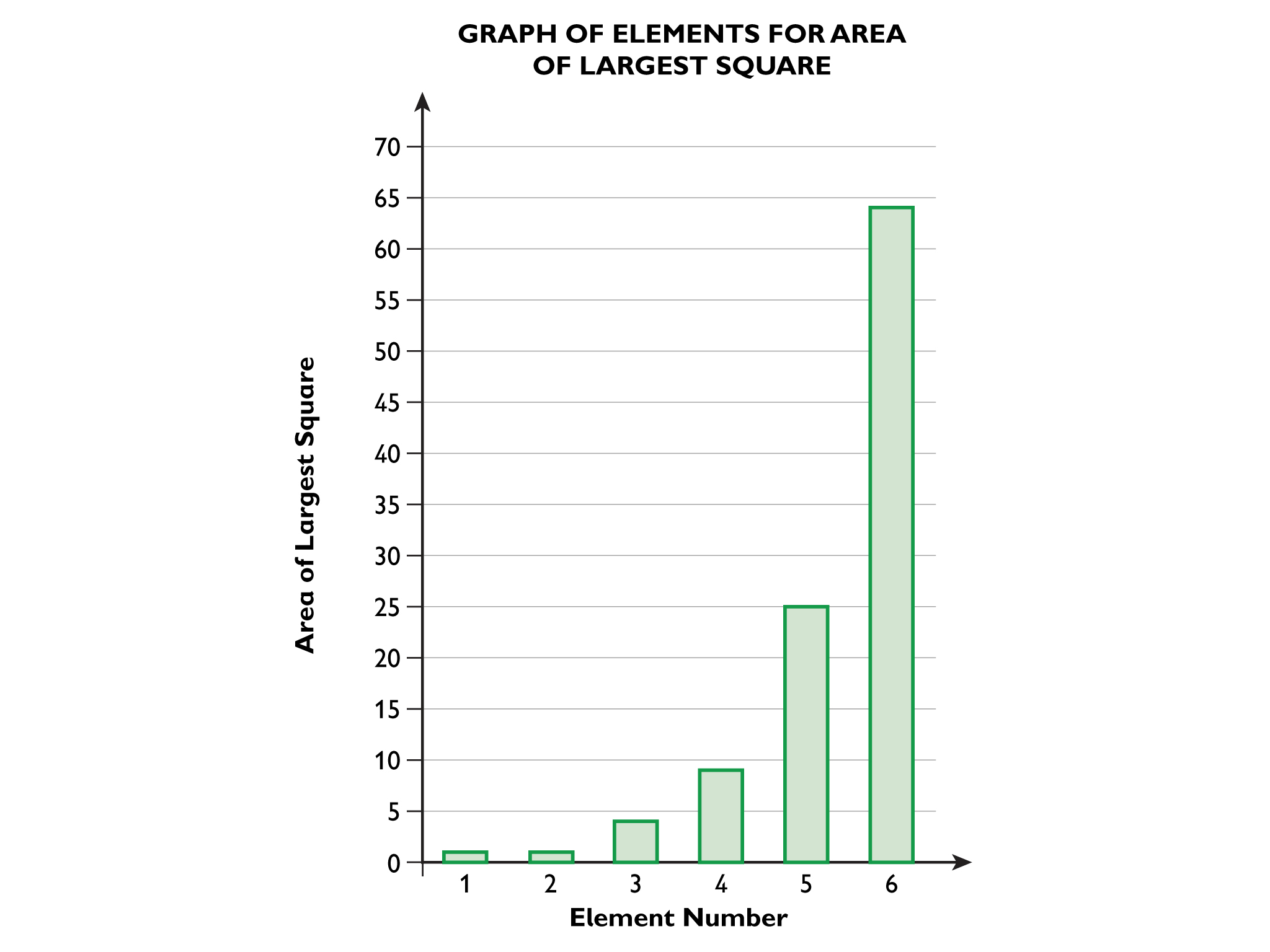4.

5. An expanding spiral.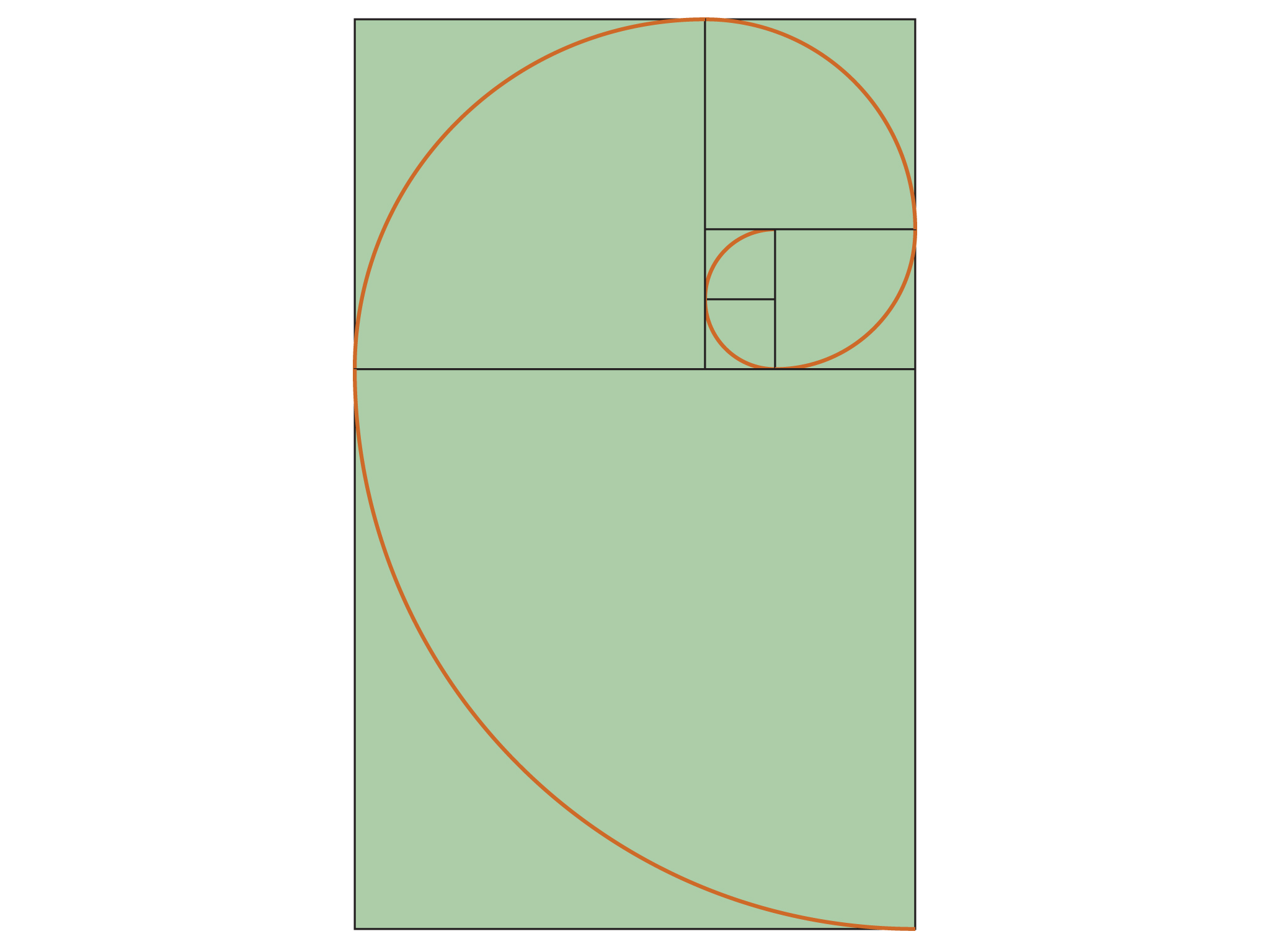Possible answer: This means that the dimensions of each chamber are proportional to one another. Meaning the ratio of the dimensions for every chamber will be the same (i.e., the Golden ratio).

7. 1, 1, 2, 3, 5, 8, 13, 21, 34, 55, 89, 144, 233, 377, 610, 987, 1 597, 2 584, 4 181, 6 765, 10 946

8. The number to the left of any two consecutive numbers is equal to the first number subtracted from the second. For example, the number to the left of 5 is equal to: 8 – 5, or 3. So the number to the left of the first 1 would be equal to 1 – 1, or 0. Continuing the sequence to the left: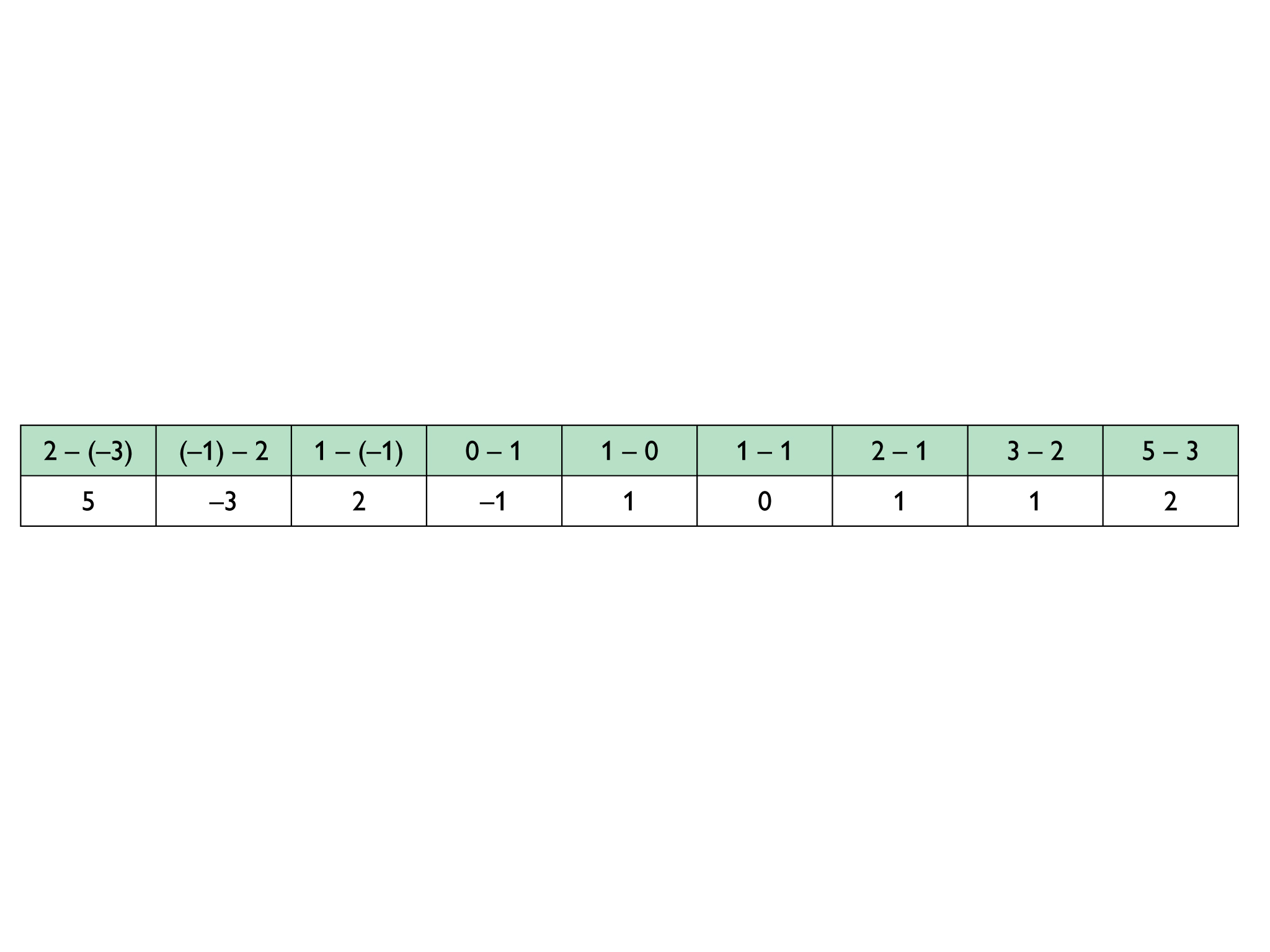You get the same series numbers in reverse order, except that every alternate number is negative.

# Spirals

You may have seen mathematical sequences with numbers like 1, 3, 5, 7, and so on. But you can also draw sequences, like these examples, where the first four elements have been drawn.

1. If the first element has side length 1, compute the dimensions (length and width) of the next elements.
2. Draw the fifth and six elements in this sequence, the fifth connecting to the left of the fourth element, and the sixth underneath the fifth element.
3. Draw a graph where the element number (1, 2, 3, …) is along the horizontal axis, and the side length of the largest square in the element (1, 1, 2, 3, …) is along the vertical axis.
4. Draw a graph where the element number (1, 2, 3, …) is along the horizontal axis and the area of the largest square in the element (1, 1, 4, …) is along the vertical axis.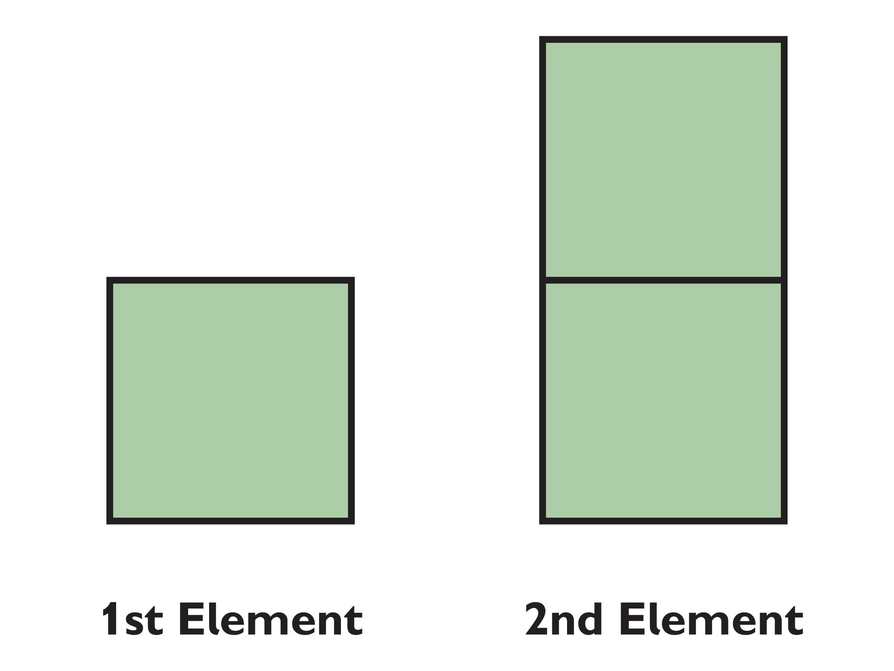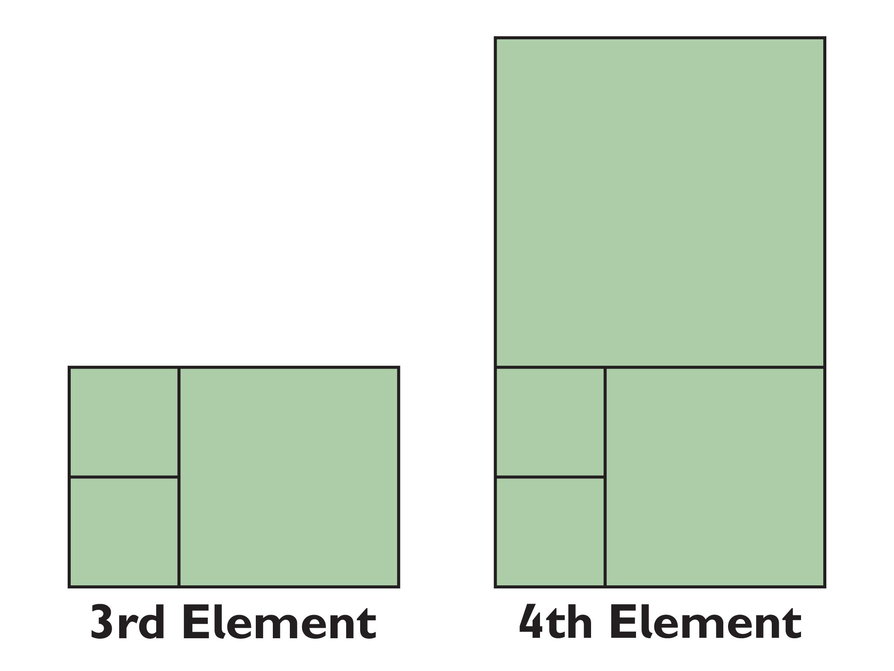5. A quarter-circle can be drawn in each new square element added to the sequence, like the images to the right. Draw the next two or three quarter-circles into your sequence. What figure seems to be shaped this way?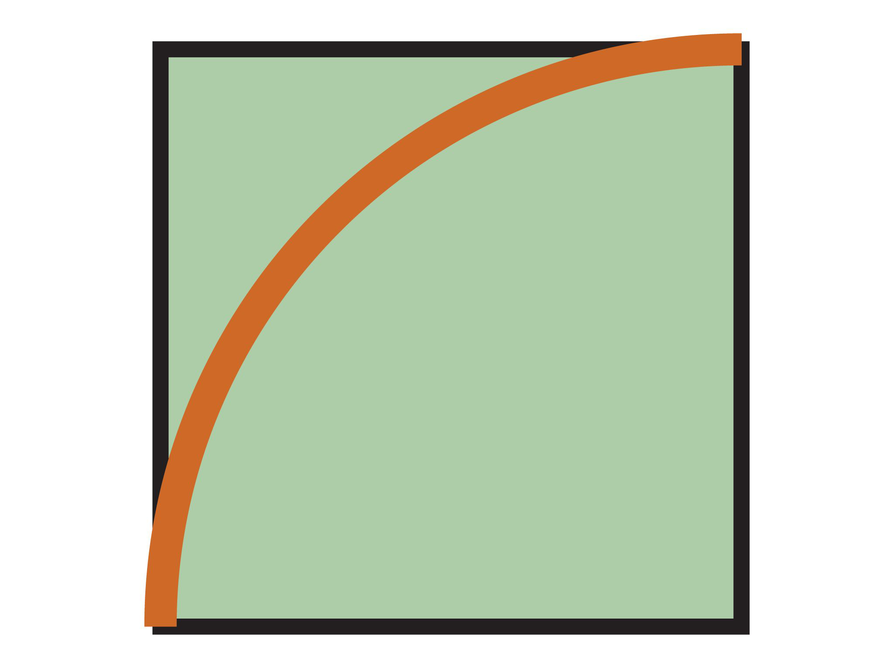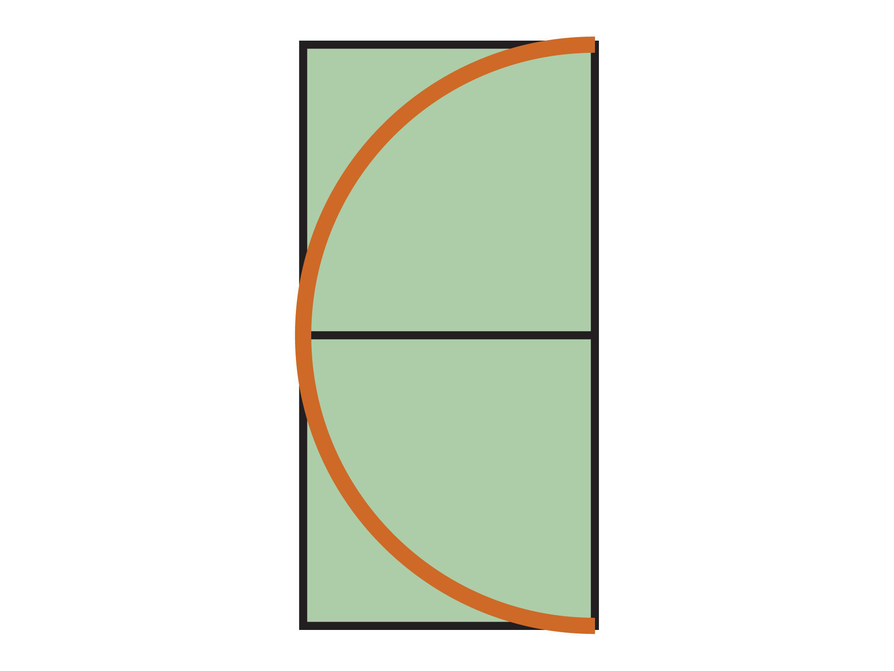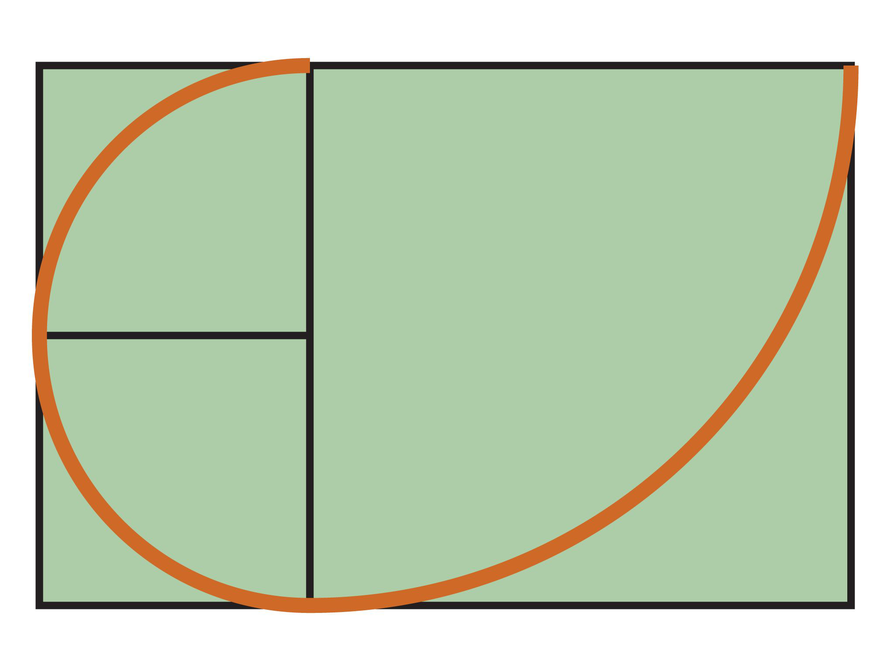6. The Fibonacci sequence is named after Leonardo of Pisa, who was known as Fibonacci. Fibonacci's book Liber Abaci, published in 1202, introduced the sequence to Western European mathematics.

The Fibonacci spiral can be found in flowers, pine cones and in the beautiful nautilus shell shown here.
The inside of a nautilus shell reveals that all chambers are more or less similar. What does that mean?

7. Complete the following sequence with another 10 elements:
1, 1, 2, …, 5, 8, …, 21, 34, …, 89, …, …
8. This sequence is called the Fibonacci sequence after an Italian mathematician. What is the general rule of the Fibonacci sequence?# Ship It!

1. Volume = width • height • length = 8 ft • 8.5 ft • 20 ft = 1,360 ft3
2. If t = volume in TEUs, and v = volume in ft3, a formula is: t = v ÷ 1,360
3. Volumes of Different Sized Cargo Containers: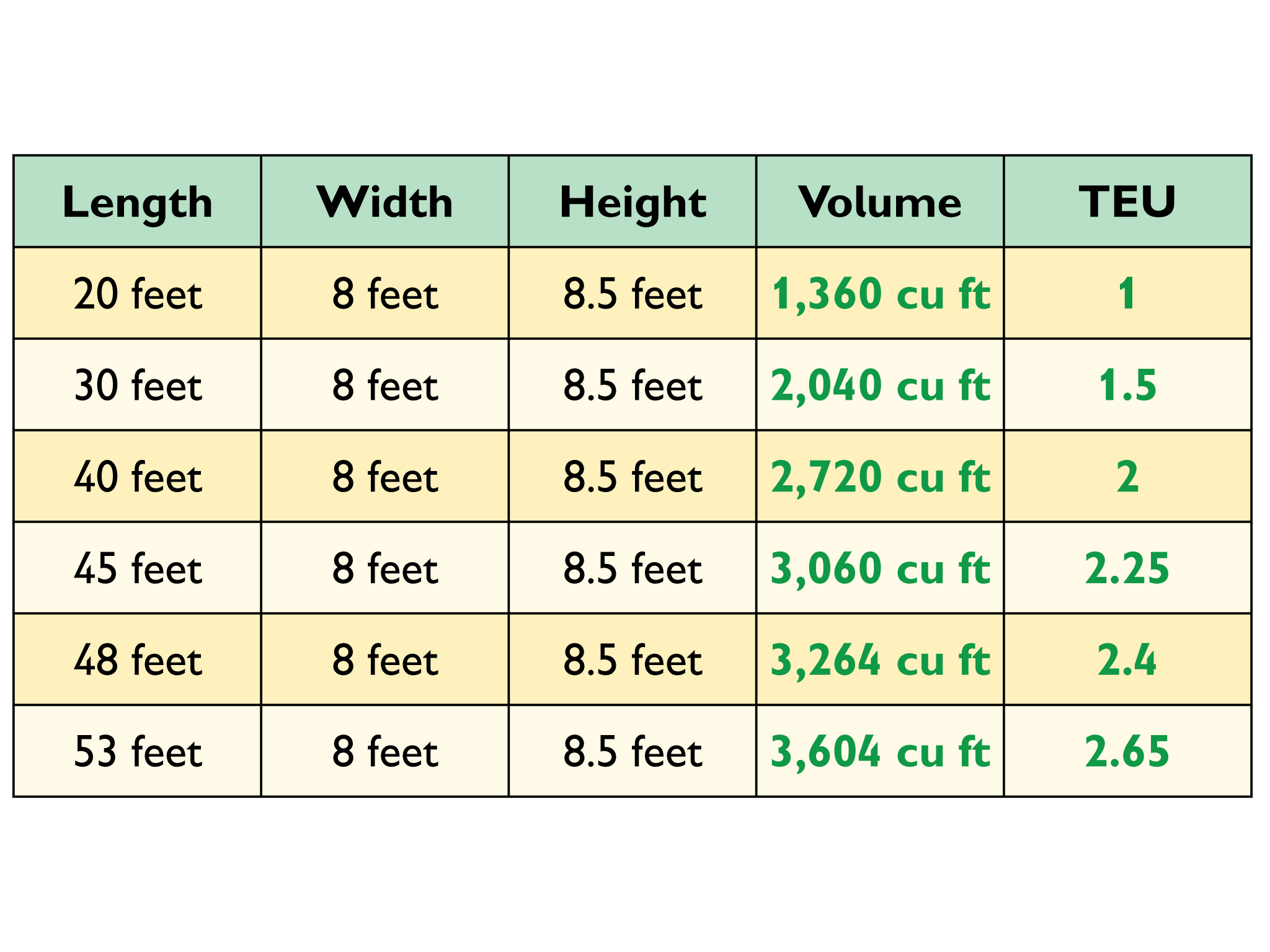4. There are 17 containers across the width of the ship. Each container is 8 feet wide. So the ship is 17 • 8 = 136 feet wide.
5. The volume is measured in TEUs probably because the numbers are much smaller, which makes calculations much easier.
6. As the containers are stacked higher, the angle between the horizon and the lowest line of sight gets narrower (smaller), and the distance from the captain to the closest point she could see increases. If the containers are stacked so high the viewing angle is 0° (horizontal), the captain will be unable to see anything on the water ahead.

# Ship It!

Many ships carry containers. Containers have fixed sizes:

Width: 8 feet

Height: 8.5 feet

Standard lengths: 20 ft, 30 ft, 40 ft, 45 ft, 48 ft, or 53 ft.

The volume of a 20 ft long container is called a TEU: twenty-foot equivalent unit.

1. What is the volume of a 20 foot container in cubic feet?
2. Write an equation that you could use to convert the volume of a container given in cubic feet to TEUs.
3. Complete the table to show the volumes of different sized containers in cubic feet and TEUs.4. In the picture to the right the containers cover the full width of the ship. Find the width of the ship.
5. Why do you think shipping companies measure volume in TEUs rather than cubic feet?
6. Look at the diagram to the lower right. How can you use your knowledge of angles to explain what happens to the line of sight of the captain as taller stacks of containers are loaded onto the ship? At what angle will the captain not be able to see any of the water directly in front of her?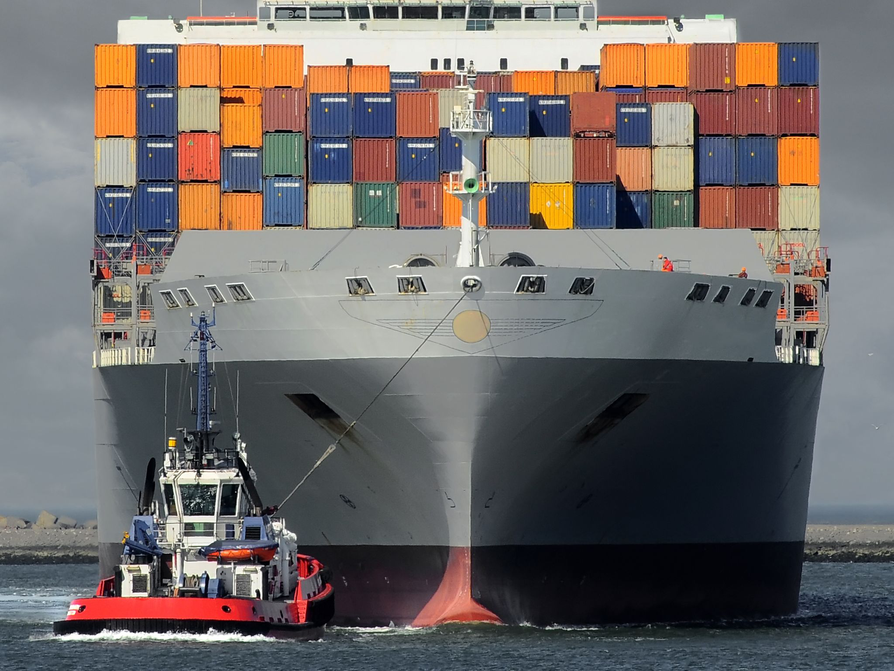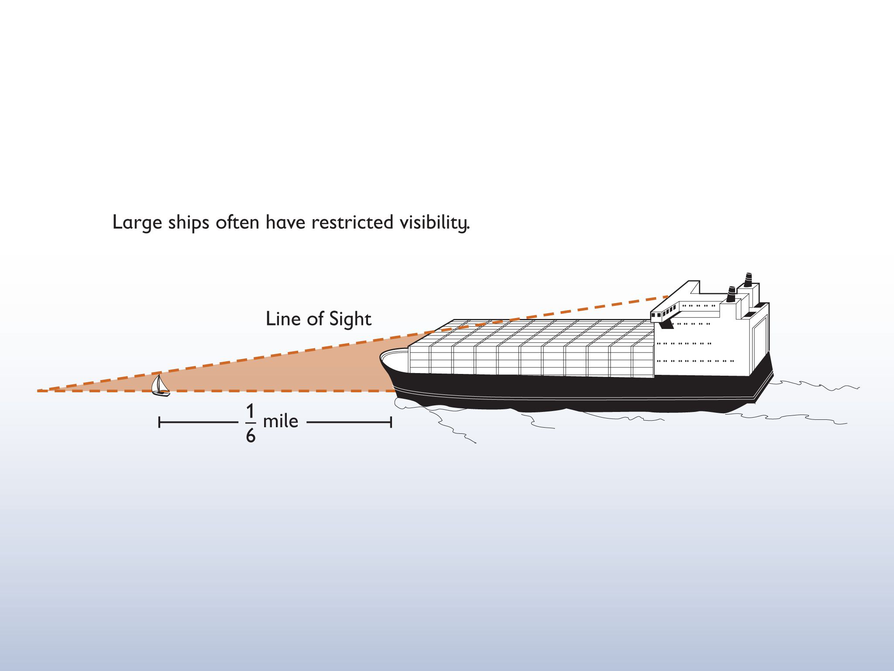HANDOUT: Ship It!

# Rideau Canal Waterway

1. The locks have a 79 feet rise over a length of 300 meters. 79 feet is approximately 23 meters.

$23\text{\hspace{0.17em}}\text{m}\text{\hspace{0.17em}}\text{rise}÷300\text{\hspace{0.17em}}\text{m}\text{\hspace{0.17em}}\text{run}\approx \text{0}\text{.08}=8\text{%}\text{\hspace{0.17em}}\text{slope}$
2. Answers will vary. The whole Welland Canal rises from 75 m to 175 m (100 m rise) over a distance of 43.5 km = 43,500 m.

100 ÷ 43,500 = approximately 0.0023 or 0.23% slope.

From locks 4 to 7, the Welland Canal rises from 117 m to 175 m (58 m rise) over a distance of 13.9 – 12.6 = 1.3 km.

1.3 km = 1,300 m

58 ÷ 1,300 = approximately 0.045 or 4.5% slope

# Rideau Canal Waterway

There is an amazing series of locks at the beginning of the Rideau Canal that goes from Ottawa, in Canada, to Lake Ontario.

Here is a map of the canal to the right.

The first 8 locks are next to the Laurier Castle, which is now a hotel.

1. The 8 locks (called a flight) bring you 79 feet up over a distance of some 300 meters. Find a way to express mathematically the steepness of these locks.

2. Compare the steepness of these locks to the locks in different sections of the Welland Canal.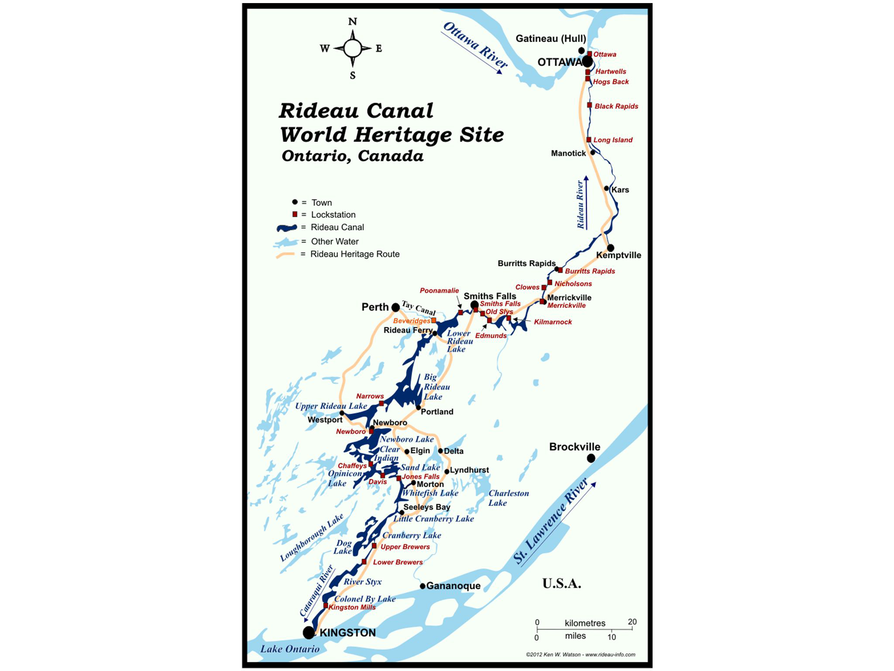# A Rule of Thumb

1. This means you can easily calculate the speed at which a train is going. For example, if you count 20 clicks in 20 seconds, then the train is going 20 mph; if you count 35 clicks in 20 seconds, the train is going 35 mph. You need to assume that the joints between rail sections are spaced evenly.

20 seconds is $\frac{1}{3}$ of a minute

$1$$\frac{1}{60}$ of an hour
So, 20 seconds is $\frac{1}{3}$ of $\frac{1}{60}$ or $\frac{1}{180}$ of an hour.

If 1 click in 20 seconds means 1 mph, that means:

• The distance between joints is $\frac{1}{180}$ of a mile long
• A mile is 5,280 feet

So, the distance between joints would need to be $\frac{5,280}{180}$, or $29\frac{1}{3}$ feet long.

# A Rule of Thumb

Railroad people use a “rule of thumb” for estimating the speed of a train you are riding on. The rule of thumb asks you to listen to the number of “clicks” the wheels makes as they cross the joints where the rails are connected.

Rule of Thumb:

The speed of a train in miles per hour is the number of clicks you count in a period of 20 seconds.

1. Explain exactly what this rule of thumb means, and explain why it works. What do you need to assume about railroad tracks?

# Intersection

1. Assuming both Broadway and Main Street continue as straight lines:

• The distance between Broadway and Main starts at 1,200 feet.
• After 700 feet, this distance has decreased by 300 feet.
• 300 is $\frac{1}{4}$ of 1,200.
• So the 700-foot distance is $\frac{1}{4}$ the whole distance before they intersect (where the distance between them becomes 0 feet).

So 700 • 4 = 2,800 feet east of Center Street is where Broadway and Main Street intersect.

# Intersection

Broadway and Main Street continue east until they intersect. Center Street and First Street are perpendicular to Main Street.

Distances given on the map are in feet.

1. How far east of Center Street does Main Street intersect Broadway?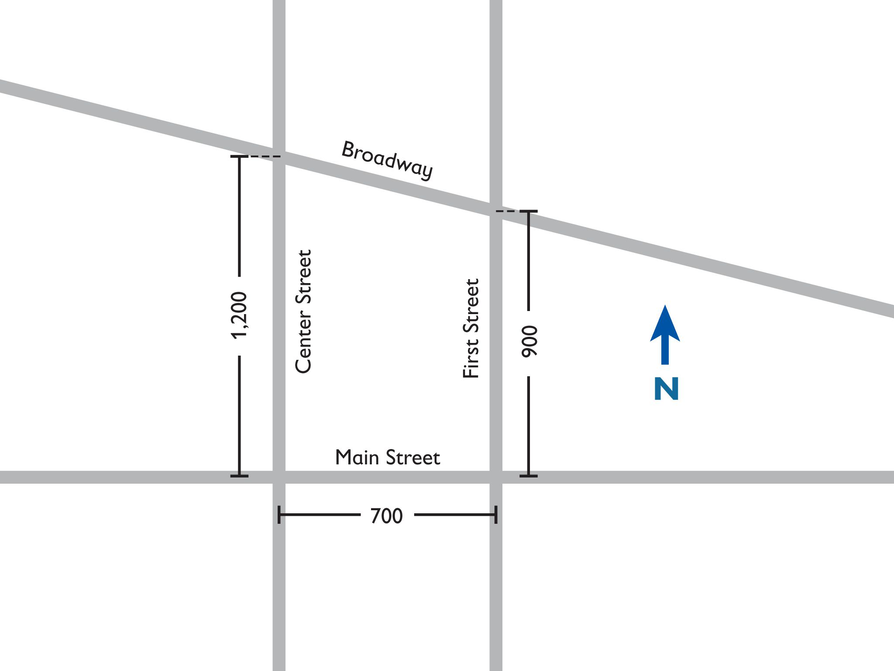# Tolstoy's Problem

1. The two meadows, the first one twice the size of the second: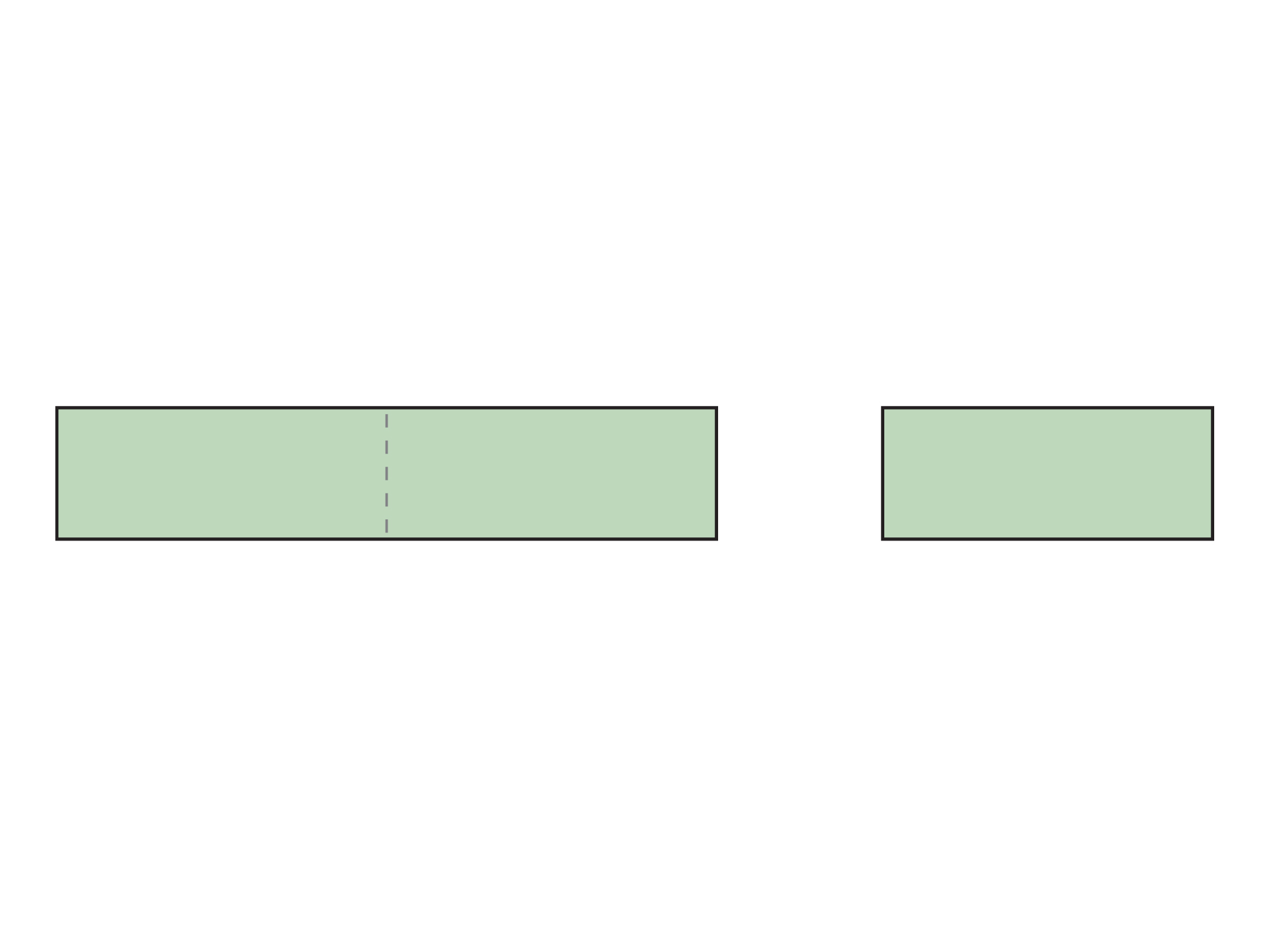The whole large meadow is scythed by the whole team working 0.5 day + one half-team working 0.5 day.

Since a half-team does $\frac{1}{2}$ the work in the same amount of time as a whole team, the work for the whole large meadow is equivalent to a half-team working 3 half-days, or $\frac{3}{4}$ of a whole team-day.

If we divide the large meadow into thirds, each third represents $\frac{1}{4}$ of a team-day.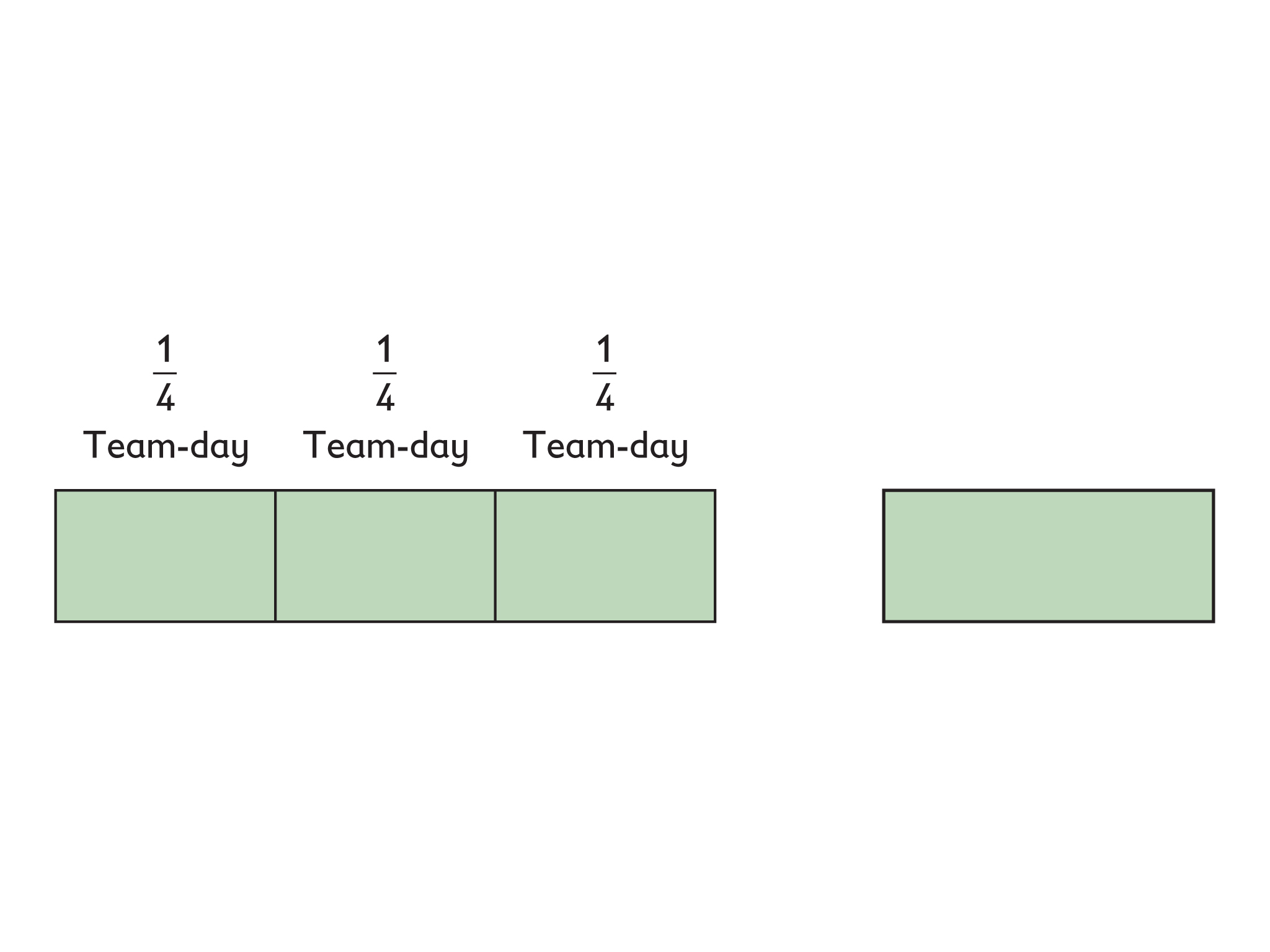In the second half of the first day, one half-team scythed part of the small meadow.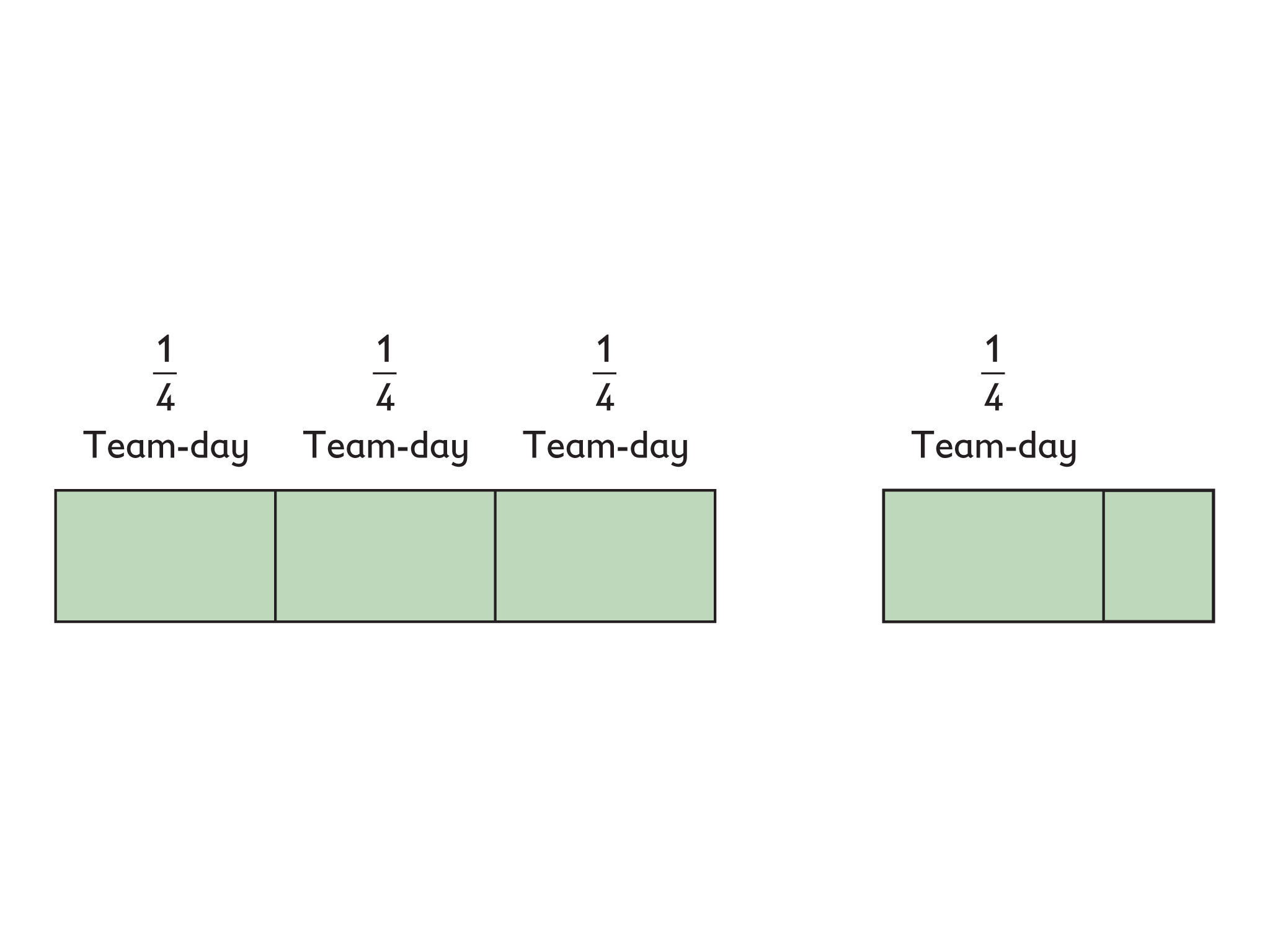Since the large meadow is 2 times the size of the small meadow, each third of the large meadow is equivalent to $\frac{2}{3}$ of the small meadow. So, the half-team scythed $\frac{2}{3}$ of the small meadow on the first day.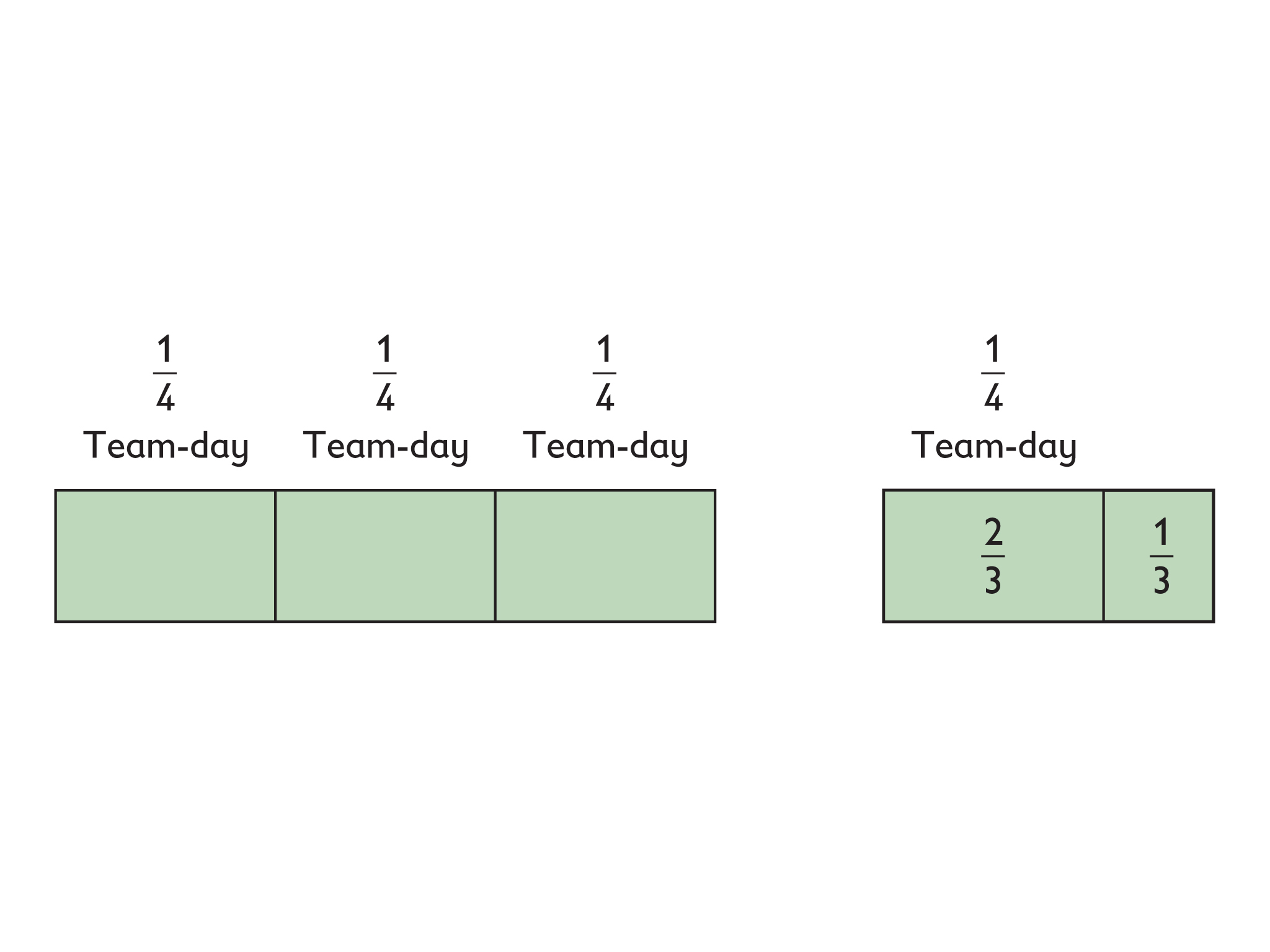The remaining third of the small meadow is scythed by one person working one day, or 1 person-day. So, the other $\frac{2}{3}$ of the small meadow is equal to 2 person-days. But it is also equal to $\frac{1}{4}$ team-day. If 2 persons are equal to $\frac{1}{4}$ team, then one whole team must be 2 • 4 = 8 people.

Answer: There were 8 people in the team of haymakers.

# Tolstoy's Problem

Leo Tolstoy posed the following problem, one he liked very much:

A team of haymakers was assigned the task of scything two meadows, one twice the size of the other. The team worked a half-day on the larger meadow. Then the team split into two equal groups. The first group remained in the larger meadow and finished scything by evening. The second group scythed the smaller meadow, but by evening there still remained a portion to do. This portion was scythed the next day by one haymaker in a single day’s work.

1. How many people were there in the team?

# The Dog Run

1. If the fence is made 10 meters long, it would only be 1 meter wide (perimeter = 10 + 10 + 1 + 1 = 22). In this case the area is 10 m2.

• If the fence is made 9 m long, it is 2 m wide, and the area is 9 • 2 = 18 m2.
• If the fence is made 6 m long, it is 5 m wide, and the area is 6 • 5 = 30 m2.
• If the fence is made 2 m long, it is 9 m wide, and the area is 2 • 9 = 18 m2.

So, a very long or very narrow dog run has a smaller area than one that is more square.

As the length and width of the fence gets closer to the same value, the area is maximized. When the length and width have a larger difference, the area is smaller.

2. Table of length, width, and area values: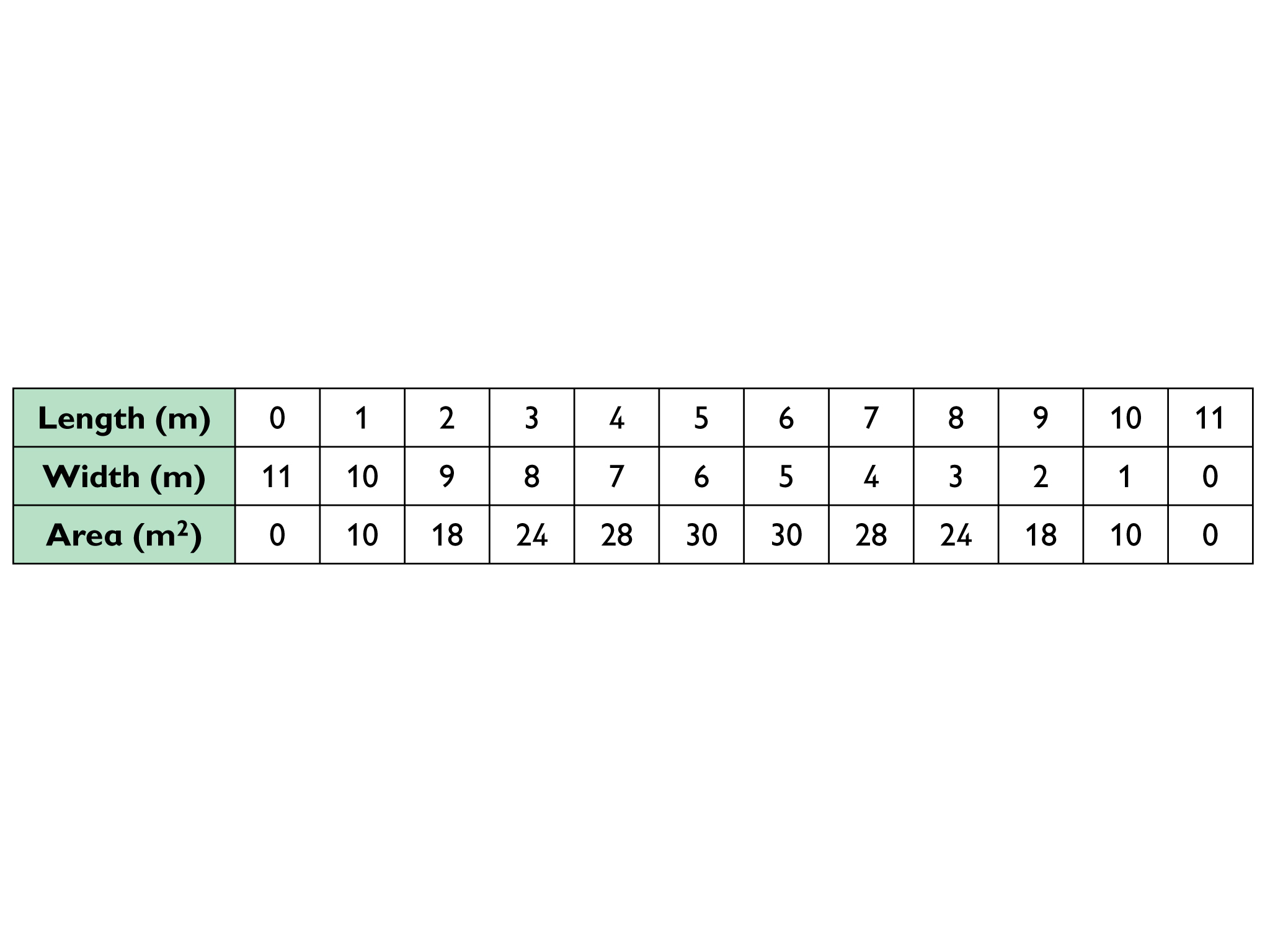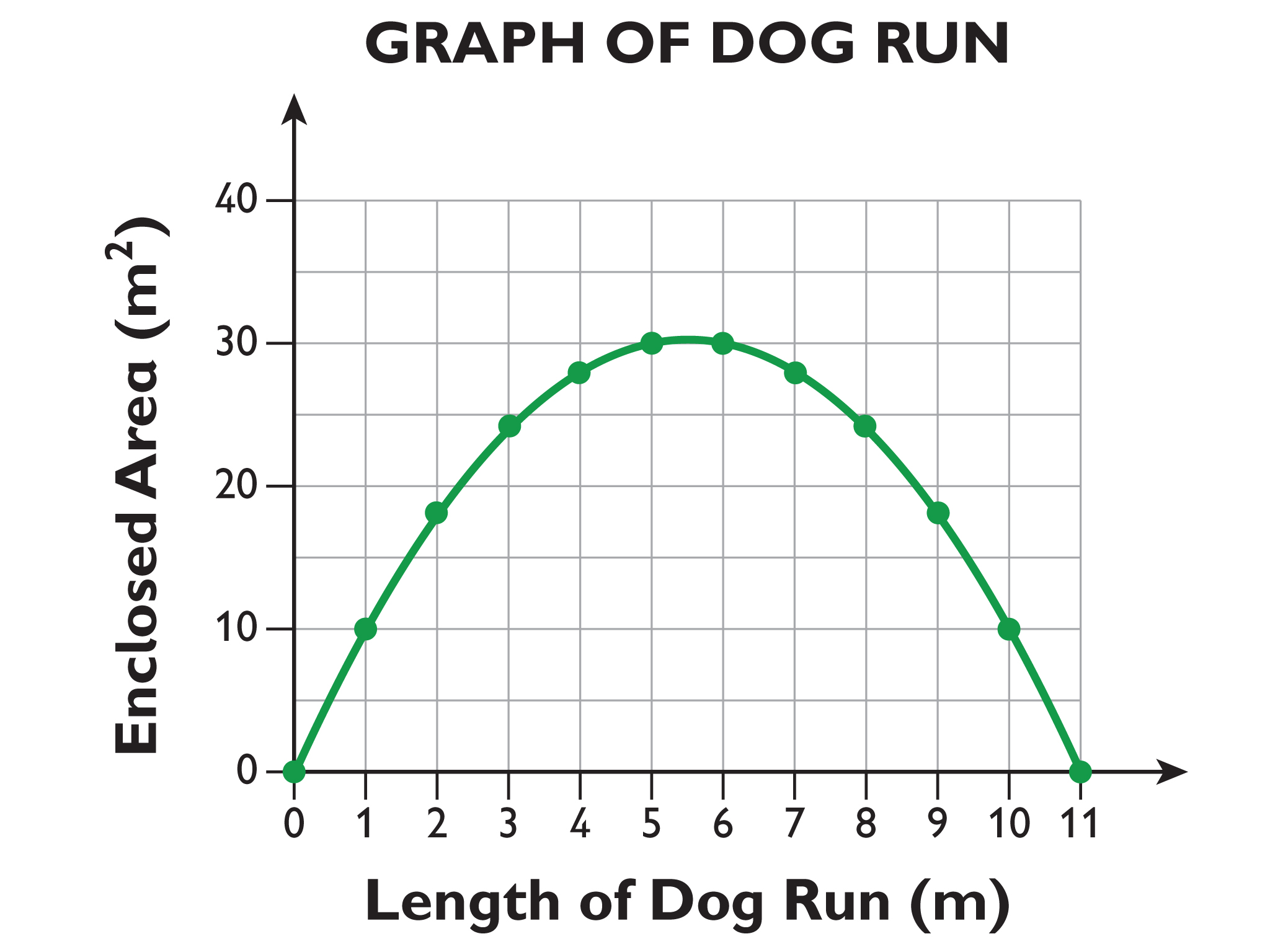# The Dog Run

A rectangular dog run is to be made from 22 meters of wire fencing. The owner is interested in knowing how the area enclosed by the fence will depend upon the length of the run.

1. Describe in writing how the enclosed area will change as the length increases through all possible values.

2. Draw a coordinate plane as the one shown below and sketch the graph illustrating your answer.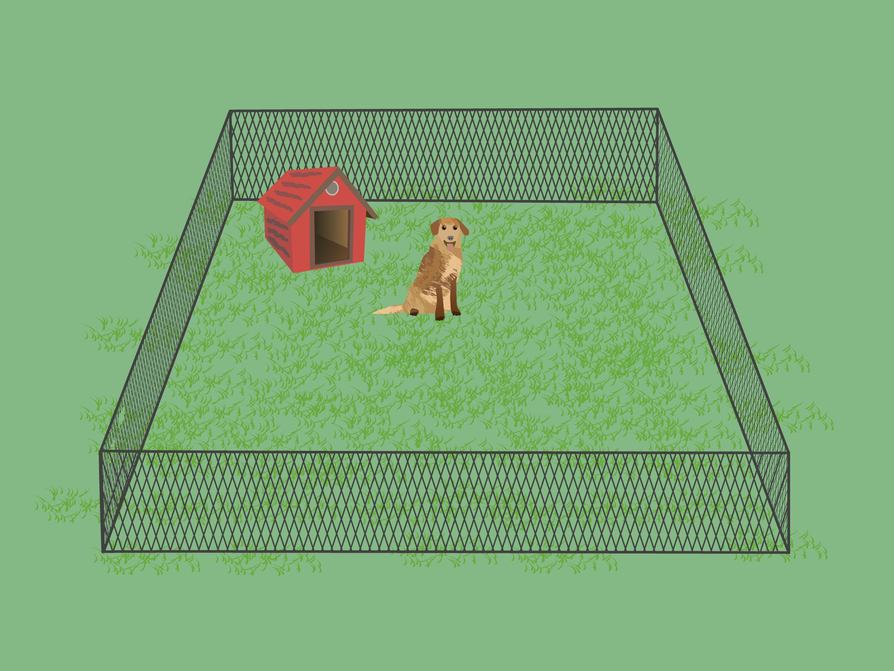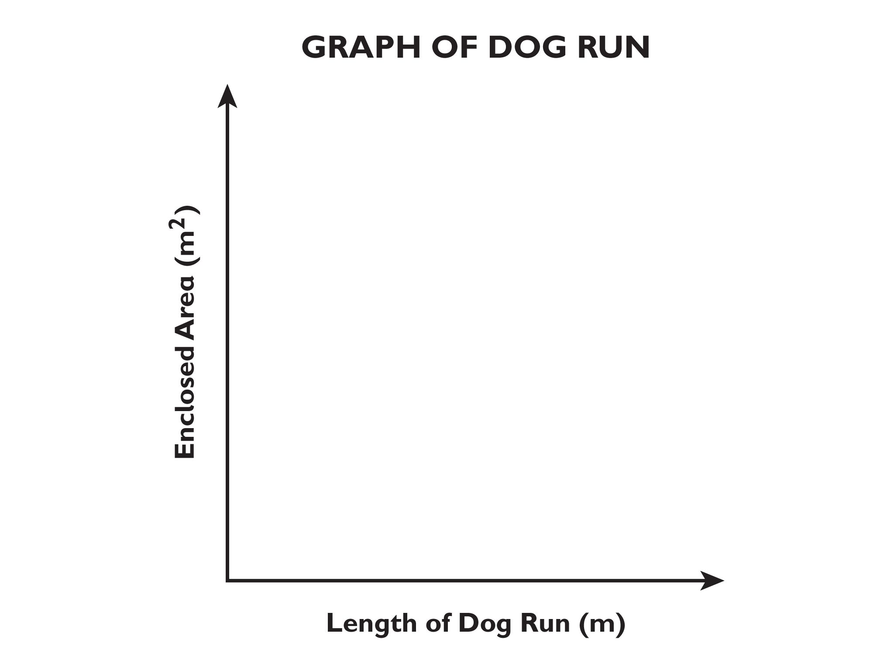# Bodies of Water

1. A correct solution:

$\begin{array}{cc}160•0.60=96& 60%\text{\hspace{0.17em}}\text{of}\text{\hspace{0.17em}}160\text{\hspace{0.17em}}\text{lb}\text{\hspace{0.17em}}\text{is}\text{\hspace{0.17em}}96\text{\hspace{0.17em}}\text{lb}.\\ \frac{12}{16}=\frac{3}{4}& \text{The}\text{\hspace{0.17em}}\text{water}\text{\hspace{0.17em}}\text{in}\text{\hspace{0.17em}}\text{each}\text{\hspace{0.17em}}\text{can}\text{\hspace{0.17em}}\text{weighs}\text{\hspace{0.17em}}\frac{3}{4}\text{\hspace{0.17em}}\text{lb}.\\ 96÷\frac{3}{4}=128& \text{There}\text{\hspace{0.17em}}\text{is}\text{\hspace{0.17em}}128\text{\hspace{0.17em}}\text{soda}\text{\hspace{0.17em}}\text{cans'}\text{\hspace{0.17em}}\text{worth}\text{\hspace{0.17em}}\text{of}\text{\hspace{0.17em}}\text{water}\text{\hspace{0.17em}}\text{in}\text{\hspace{0.17em}}96\text{\hspace{0.17em}}\text{lb}.\end{array}$

So, 120 is a reasonable approximation; however, “about 130” or “between 125 and 130” would be closer, and “128” would be more precise.

# Bodies of Water

According to the Runner’s World website:

• On average, the human body is more than 50 percent water [by weight]. Runners and other endurance athletes average around 60 percent. This equals about 120 soda cans’ worth of water in a 160-pound runner!
• A typical soda can holds 12 fluid ounces. One pint (16 fluid ounces) of water weighs one pound.
1. Are there really about 120 soda cans’ worth of water in the body of a 160-pound runner? Show each step of your work.